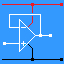RANDOM PAGE

SITE SEARCH

LOG
IN

HELP

# Simplifying Logic

This is the AQA version closing after June 2019. Visit the the version for Eduqas instead.

## Techniques

If you have a good understanding of the logic gate rules, it is possible to work out a simpler solution just by examining the truth table and thinking hard. But this is a rather hit and miss approach and Karnaugh maps and Boolean algebra can be faster and more reliable methods but neither is easy.

Boolean algebra can be used to simplify expressions/circuits to generate a more optimal solution.

## Logic Operations

• + means OR
• . means AND
• a line across the top means NOT
• ⊕ means XOR## De Morgan

• A + B = A . B• A . B = A + B## De Morgan

• A + B = A . B
• A . B = A + B

## Gate Behaviour

• AND GATE
• A . 1 = A        The gate is open.
• A . 0 = 0        The gate is closed - output low.
• OR GATE
• A + 1 = 1        The gate is closed - output high.
• A + 0 = A        The gate is open.

## Commutative

• When using AND and OR, it makes no difference if the inputs are swapped.
• A . B = B . A
• A + B = B + A

## Associative

• The presence or absence of brackets makes no difference.
• A . (B . C) = (A . B) . C = A . B . C
• A + (B + C) = (A + B) + C = A + B + C

## Distributive

• A . (B + C) = A . B + A . C
• (A + B) . (A + C) = A + (B . C)

## Identity

• A + A = A
• A . A = A
• A . B + A . B = A
• (A + B) . ( A + B) = A

## Redundancy

In all these expressions, one of the items has no effect and can be removed.

• A . (A + B) = A
• A + A . B = A
• 0 . A = 0
• 0 + A = A
• 1 . A = A
• 1 + A = 1
• A . A = 0
• A + A = 1
• A . (A + B) = A . B
• A + A . B = A + B

reviseOmatic V3     Contacts, ©, Cookies, Data Protection and Disclaimers Hosted at linode.com, London